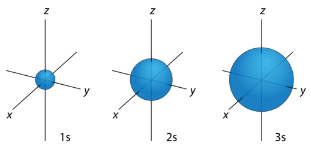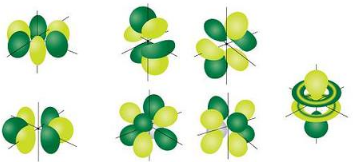# 5.13: Orbitals

$$\newcommand{\vecs}{\overset { \rightharpoonup} {\mathbf{#1}} }$$ $$\newcommand{\vecd}{\overset{-\!-\!\rightharpoonup}{\vphantom{a}\smash {#1}}}$$$$\newcommand{\id}{\mathrm{id}}$$ $$\newcommand{\Span}{\mathrm{span}}$$ $$\newcommand{\kernel}{\mathrm{null}\,}$$ $$\newcommand{\range}{\mathrm{range}\,}$$ $$\newcommand{\RealPart}{\mathrm{Re}}$$ $$\newcommand{\ImaginaryPart}{\mathrm{Im}}$$ $$\newcommand{\Argument}{\mathrm{Arg}}$$ $$\newcommand{\norm}{\| #1 \|}$$ $$\newcommand{\inner}{\langle #1, #2 \rangle}$$ $$\newcommand{\Span}{\mathrm{span}}$$ $$\newcommand{\id}{\mathrm{id}}$$ $$\newcommand{\Span}{\mathrm{span}}$$ $$\newcommand{\kernel}{\mathrm{null}\,}$$ $$\newcommand{\range}{\mathrm{range}\,}$$ $$\newcommand{\RealPart}{\mathrm{Re}}$$ $$\newcommand{\ImaginaryPart}{\mathrm{Im}}$$ $$\newcommand{\Argument}{\mathrm{Arg}}$$ $$\newcommand{\norm}{\| #1 \|}$$ $$\newcommand{\inner}{\langle #1, #2 \rangle}$$ $$\newcommand{\Span}{\mathrm{span}}$$Figure $$\PageIndex{1}$$ (Credit: User:Baseball Bugs/Wikimedia Commons; Source: http://commons.wikimedia.org/wiki/File:NWA_Plane_over_Lake_Harriet_020623.JP; License: Public Domain)

### How is it that so many planes are able to fly without running into each other?

The flight path of a commercial airliner is carefully regulated by the Federal Aviation Administration. Each airplane must maintain a distance of five miles from another plane flying at the same altitude, and be 2,000 feet above and below another aircraft (1,000 feet if the altitude is less than 29,000 feet). So, each aircraft only has certain positions it is allowed to maintain while it flies. Quantum mechanics demonstrates that electrons have similar restrictions on their locations.

## Orbitals

We can apply our knowledge of quantum numbers to describe the arrangement of electrons for a given atom. We do this with something called electron configurations. They are effectively a map of the electrons for a given atom. We look at the four quantum numbers for a given electron and then assign that electron to a specific orbital.

## $$s$$ Orbitals

For any value of $$n$$, a value of $$l=0$$ places that electron in an $$s$$ orbital. This orbital is spherical in shape:Figure $$\PageIndex{2}$$ (Credit: Christopher Auyeung; Source: CK-12 Foundation; License: CC BY-NC 3.0)

## $$p$$ Orbitals

From the table below, it is evident that there are three possible orbitals when $$l=1$$. These are designated as $$p$$ orbitals and have dumbbell shapes. Each of the $$p$$ orbitals has a different orientation in three-dimensional space.Figure $$\PageIndex{3}$$ (Credit: Christopher Auyeung; Source: CK-12 Foundation; License: CC BY-NC 3.0)

## $$d$$ Orbitals

When $$l=2$$, $$m_l$$ values can be $$-2, \: -1, \: 0, \: +1, \: +2$$ for a total of five $$d$$ orbitals. Note that all five of the orbitals have specific three-dimensional orientations.Figure $$\PageIndex{4}$$ (Credit: Christopher Auyeung; Source: CK-12 Foundation; License: CC BY-NC 3.0)

## $$f$$ Orbitals

The most complex set of orbitals are the $$f$$ orbitals. When $$l=3$$, $$m_l$$ values can be $$-3, \: -2, \: -1, \: 0, \: +1, \: +2, \: +3$$ for a total of seven different orbital shapes. Again, note the specific orientations of the different $$f$$ orbitals.Figure $$\PageIndex{5}$$ (Credit: Christopher Auyeung; Source: CK-12 Foundation; License: CC BY-NC 3.0)
Table $$\PageIndex{1}$$: Electron Arrangement Within Energy Levels
Principal Quantum Number $$\left( n \right)$$ Allowable Sublevels Number of Orbitals per Sublevel Number of Orbitals per Principal Energy Level Number of Electrons per Sublevel Number of Electrons per Principal Energy Level
1 $$s$$ 1 1 2 2
2 $$s$$ 1 4 2 8
$$p$$ 3 6
3 $$s$$ 1 9 2 18
$$p$$ 3 6
$$d$$ 5 10
4 $$s$$ 1 16 2 32
$$p$$ 3 6
$$d$$ 5 10
$$f$$ 7 14

## Summary

• There are four different classes of electron orbitals.
• Electron orbitals are determined by the value of the angular momentum quantum number $$l$$.

## Review

1. What is an electron configuration?
2. How many electrons are in the $$n=1$$ orbital?
3. What is the total number of electrons in a $$p$$ orbital?
4. How many electrons does it take to completely fill a $$d$$ orbital?

5.13: Orbitals is shared under a CK-12 license and was authored, remixed, and/or curated by CK-12 Foundation via source content that was edited to conform to the style and standards of the LibreTexts platform; a detailed edit history is available upon request.